Home Practice
For learners and parents For teachers and schools
Textbooks
Full catalogue
Pricing SupportLog in

We think you are located in United States. Is this correct?

# 12.7 Representing data

## 12.7 Representing data (EMG76)

Once a research question has been developed, we collect data. The next step is to classify and organise the data. This is then followed by summarizing the data using the measures of central tendency and spread. More importantly the data that has been collected, classified, organised and summarised needs to be represented and interpreted graphically. The following different types of graphical representations will be explained in this section: pie charts, histograms, single bar graphs, line graphs and broken line graphs.

### Pie charts (EMG77)

Pie charts are best to use when you are trying to compare parts of a whole. They do not show changes over time. A pie chart is divided into sectors, usually labeled as percentages. Each sector represents a particular proportion of the data. Information can be easily read from a pie chart but contrary to that, it is difficult to identify the trend. This means that although it may be possible to use a pie chart to show the monthly rainfall of a particular town, it would be difficult to identify trends in the rainfall pattern.

## Understanding pie charts

Exercise 12.5

Consider the pie chart below and answer the questions that follow: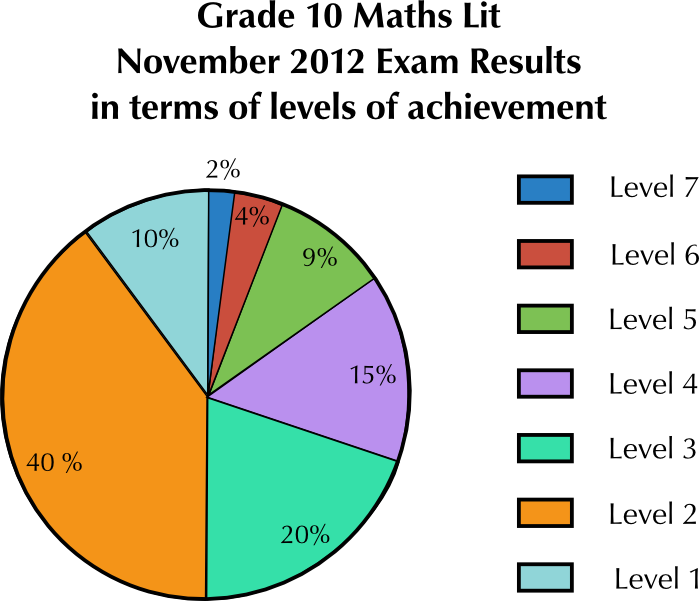Which level did most of the learners obtain?

Level $$\text{2}$$

What was the percentage of learners who obtained this level?

$$\text{40}$$ percent of learners obtained this level

Few learners achieved level $$\text{7}$$. What was the percentage of learners at this level?

$$\text{2}\%$$

If there were $$\text{120}$$ learners who wrote the examination, how many learners achieved level $$\text{4}$$?

$$\text{15}\%$$ of $$\text{120}$$ learners = $$\text{18}$$ learners who obtained level $$\text{4}$$

Write the ratio of learners who achieved level $$\text{3}$$ to those at level $$\text{2}$$ in its simplest form.

$$\text{20}$$ : $$\text{40}$$ = $$\text{1}$$ : $$\text{2}$$.

### Bar graphs (EMG78)

Bar graphs are used to display data that compared in categories. For example, every month for five months, a new store keeps count of how many customers visit the store. We can represent this on a bar graph as shown below: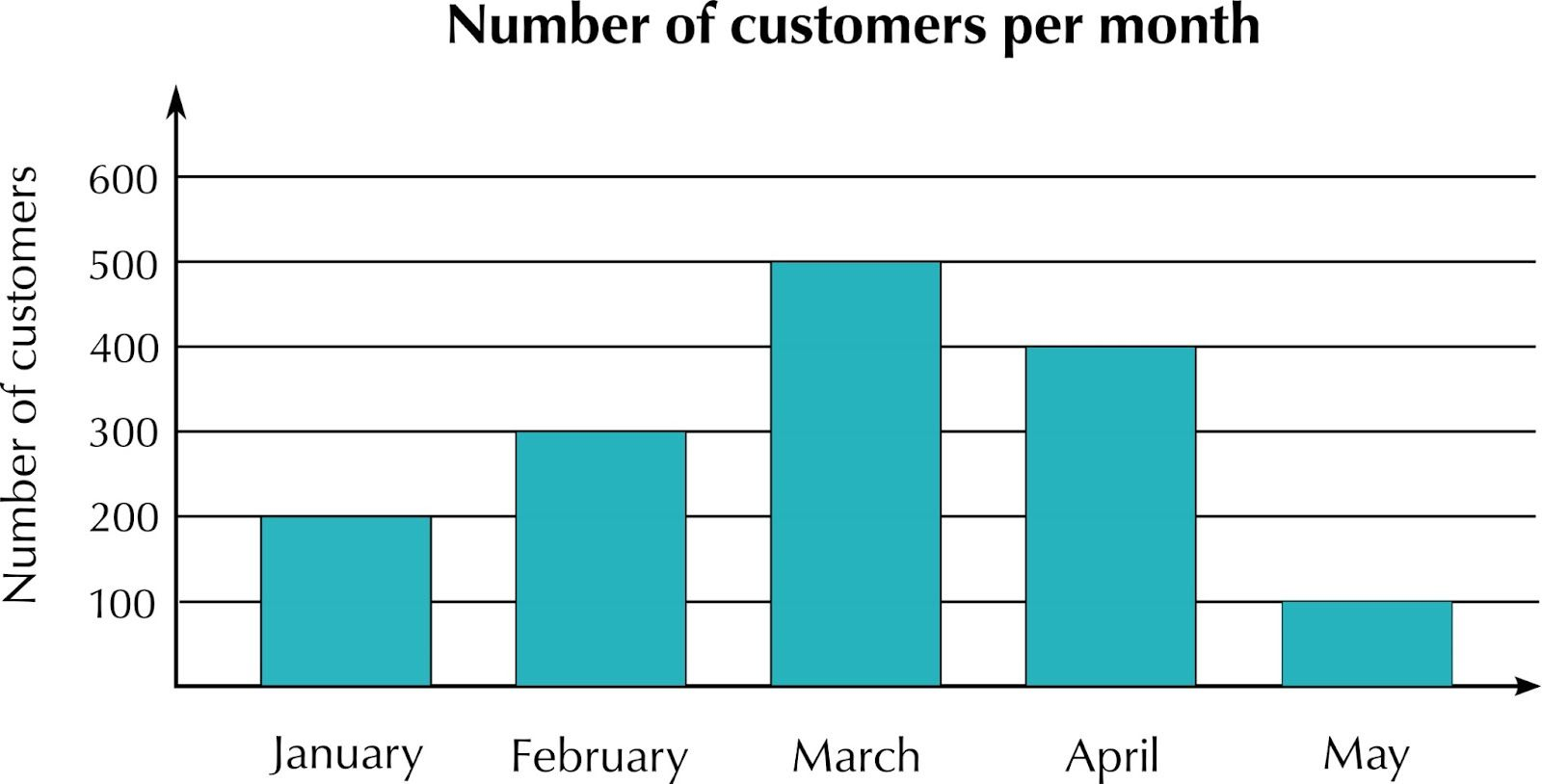### Histograms (EMG79)

Histograms are different from bar graphs in that they usually represent continuous data. Data that is displayed on a histogram is also grouped. Consider the following table of the foot lengths of learners in a class, and the frequency of those lengths.

 Foot length Frequency $$\text{22}$$ - $$\text{23,9}$$ $$\text{cm}$$ $$\text{3}$$ $$\text{24}$$ - $$\text{25,9}$$ $$\text{cm}$$ $$\text{10}$$ $$\text{26}$$ - $$\text{27,9}$$ $$\text{cm}$$ $$\text{8}$$ $$\text{28}$$ $$\text{cm}$$ and longer $$\text{4}$$

We can represent this information on a histogram as follows: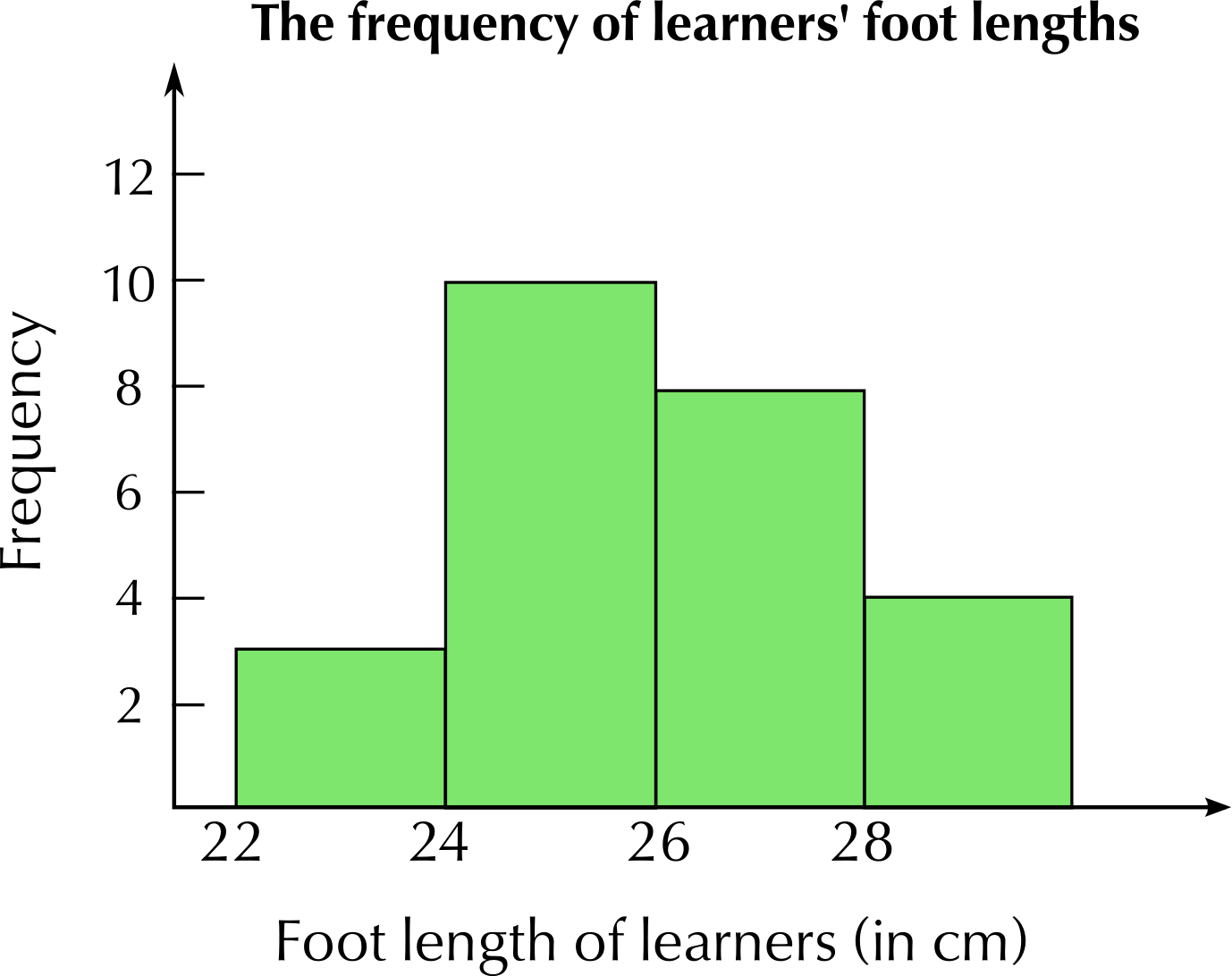Notice that unlike in the bar graph in the previous section, in the histogram, the bars are drawn next to each other - there are no spaces between them. This is to indicate that the data is continuous.

## Worked example 7: Drawing bar graphs and histograms

1. The school tuckshop keeps track of how many hot dogs, sandwiches, salads and burgers they sell at one break time. They have the data given in the table below. Draw a bar graph to represent this data.
 Item Frequency Hot dogs $$\text{15}$$ Sandwiches $$\text{35}$$ Salads $$\text{10}$$ Burgers $$\text{12}$$
2. Lwanda measures the length of his school books (in cm) and draws up the frequency table below. Draw a histogram to represent this data.
 Length of Book Frequency $$\text{20}$$ - $$\text{23,9}$$ $$\text{cm}$$ $$\text{4}$$ $$\text{24}$$ - $$\text{26,9}$$ $$\text{cm}$$ $$\text{7}$$ $$\text{27}$$ - $$\text{29,9}$$ $$\text{cm}$$ $$\text{5}$$ Longer than $$\text{30}$$ $$\text{cm}$$ $$\text{3}$$
1.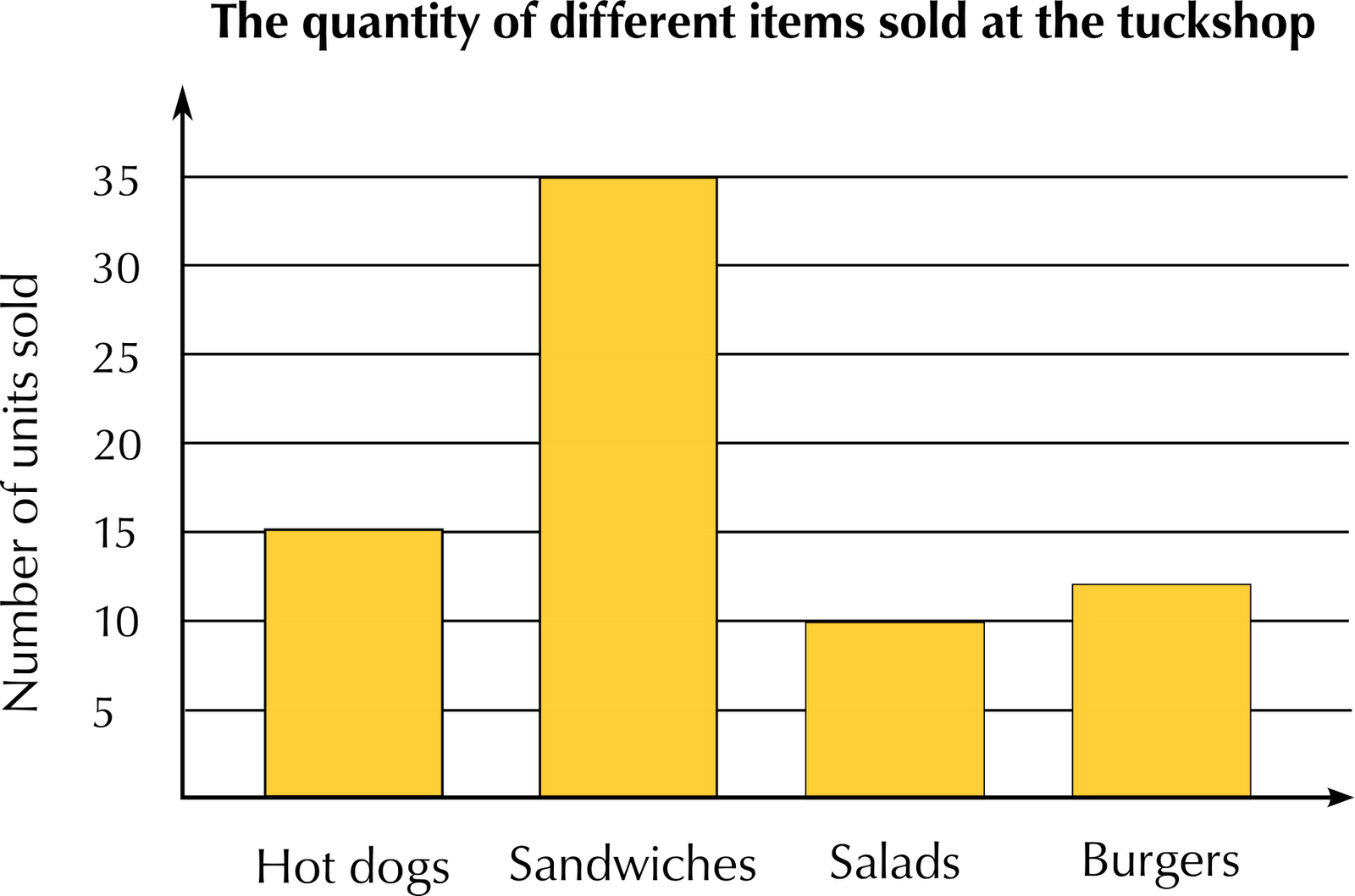2.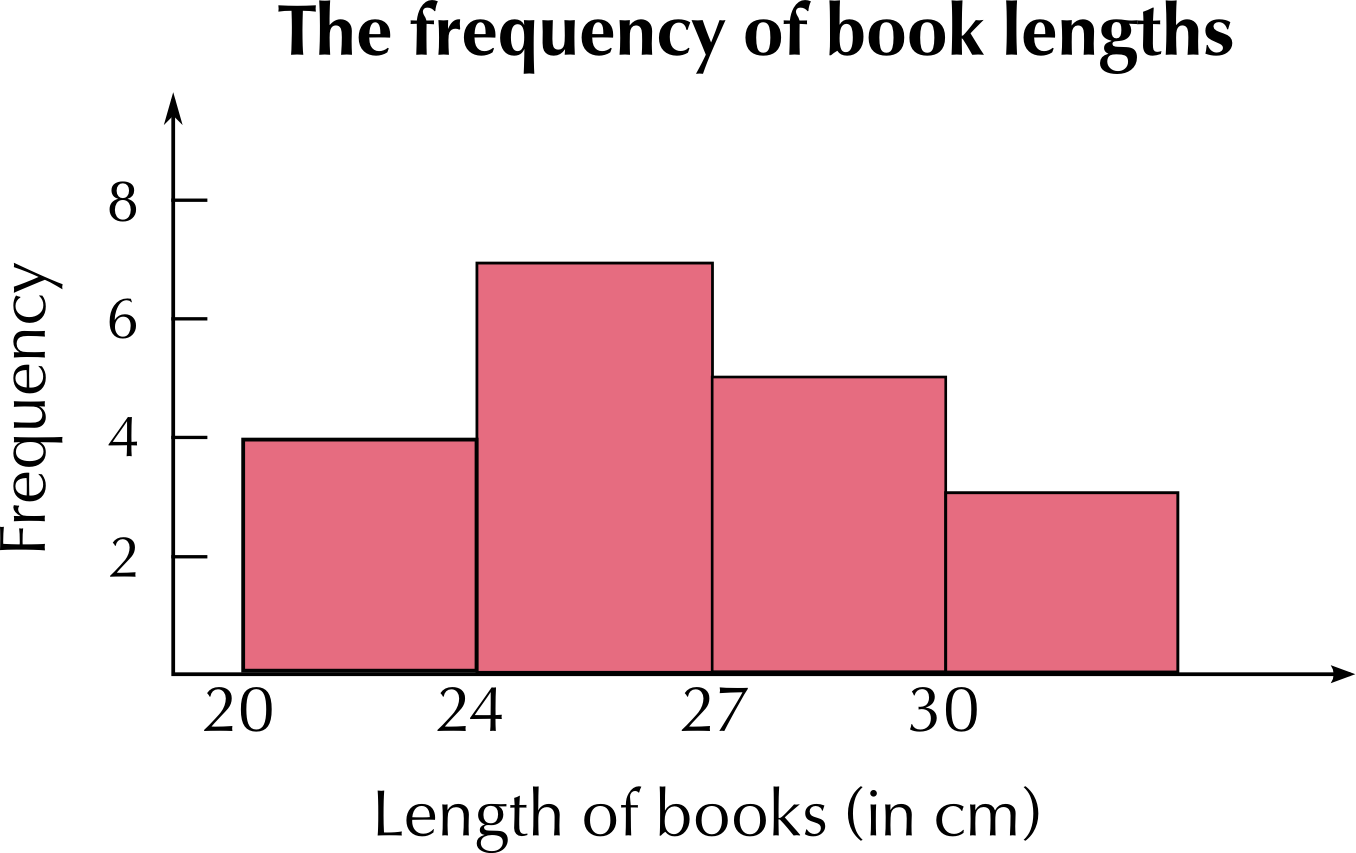Note from the previous worked example that when we draw both bar graphs and histograms, it is important to use an appropriate interval for the vertical axis. For example, using an interval of $$\text{100}$$ would not be appropriate if our largest frequency is only $$\text{15}$$, and using an interval of $$\text{1}$$ would not be appropriate if we had a maximum frequency of $$\text{500}$$ - it would make our graph very large and hard to read!

### Line graphs (EMG7B)

In data handling we use line graphs to show the relationship between two quantities. The horizontal axis often represents time, as these kinds of graphs are particularly useful for showing changes over time.

For example, we can plot the manner in which the temperature of water in a pot being heated, increases, where the temperature is taken every $$\text{30}$$ seconds.Time ($$\text{30}$$ second intervals) $$\text{0}$$ $$\text{30}$$ $$\text{60}$$ $$\text{90}$$ Temperature (°C) $$\text{20}$$ $$\text{40}$$ $$\text{60}$$ $$\text{80}$$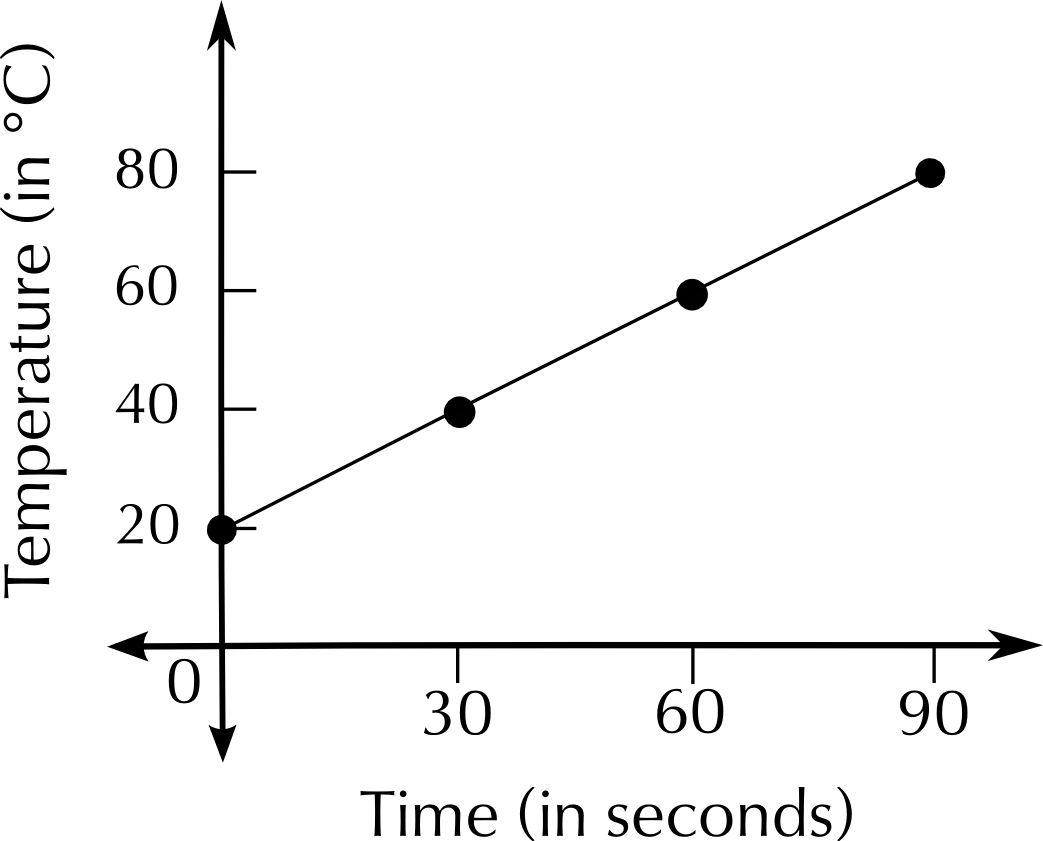## Worked example 8: Representing data on a line graph

This table below shows the average number of minutes that Jabu spent watching TV from January to November last year.

 Month Jan Feb Mar Apr May Jun Jul Aug Sep Oct Nov Daily TV viewing time (min) $$\text{108}$$ $$\text{103}$$ $$\text{108}$$ $$\text{120}$$ $$\text{115}$$ $$\text{122}$$ $$\text{116}$$ $$\text{105}$$ $$\text{110}$$ $$\text{105}$$ $$\text{104}$$1. Plot this data on a set of axes.
2. Can you observe any trends or patterns in the data? Give some possible reasons for these trends.
3. Would you be able to represent this data in a bar graph?
4. What is the advantage of using a line graph to show this information?
1. The points are plotted and connected with line segments.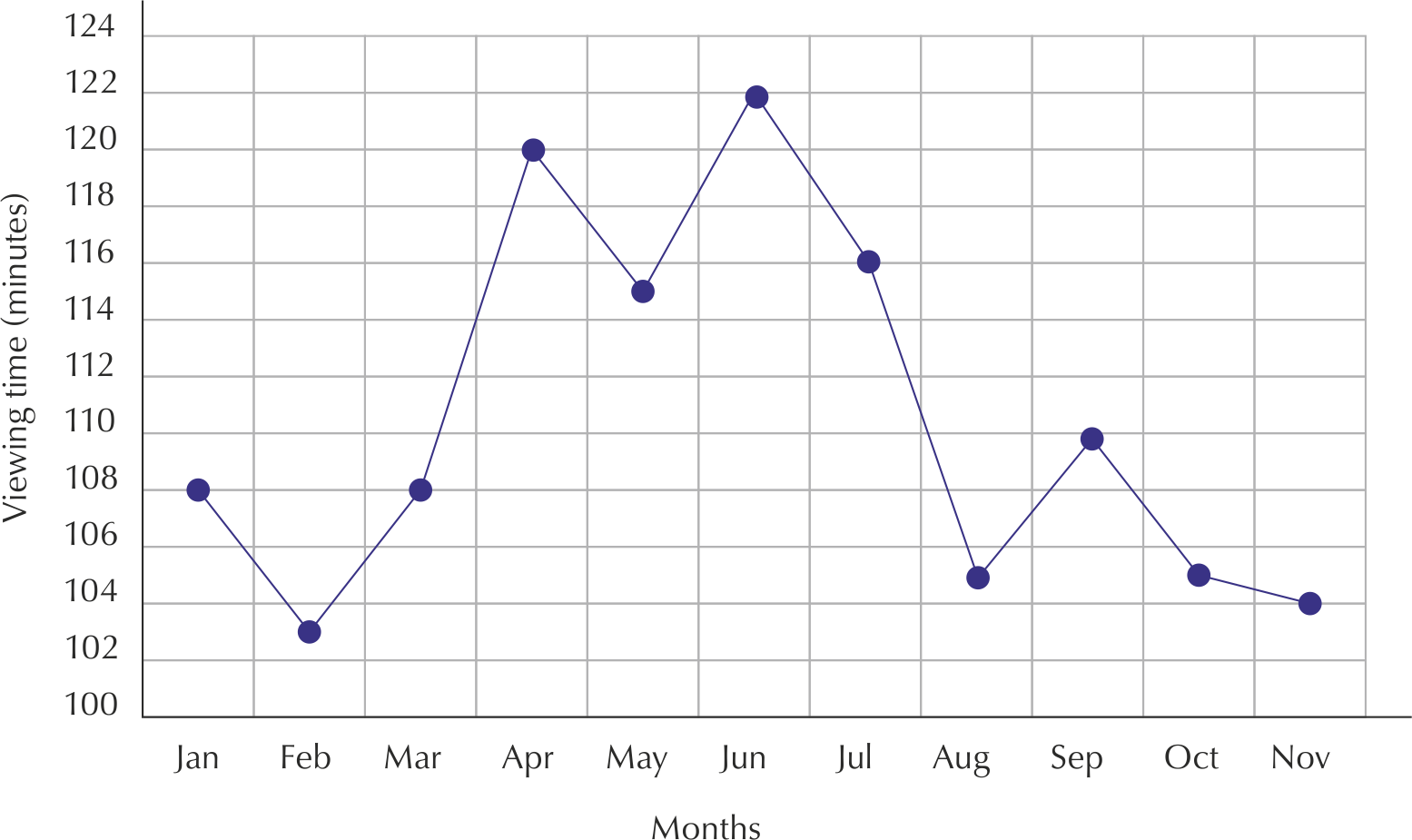1. You can see that Jabu's viewing time increases in April and again in June and slightly in September (perhaps due to school holidays). We also see decreases in his viewing time during February, May, August, October and November. These could be times when he was preparing for tests and exams.
2. Yes, it would be possible to represent this data in a bar graph; the number of minutes would be plotted as a bar for each month.
3. A line graph helps us to see trends because we can easily see the increasing or decreasing slope of each line segment in the graph.

## Representing data

Exercise 12.6

There are $$\text{300}$$ learners at a school sports day. There are four sports teams, represented by red, blue, green and yellow. Someone records the colours of the T-shirts the learners are wearing.

 Colour of T-shirt Red Blue Green Yellow Frequency $$\text{75}$$ $$\text{93}$$ $$\text{78}$$ $$\text{54}$$

Represent this data in a pie chart.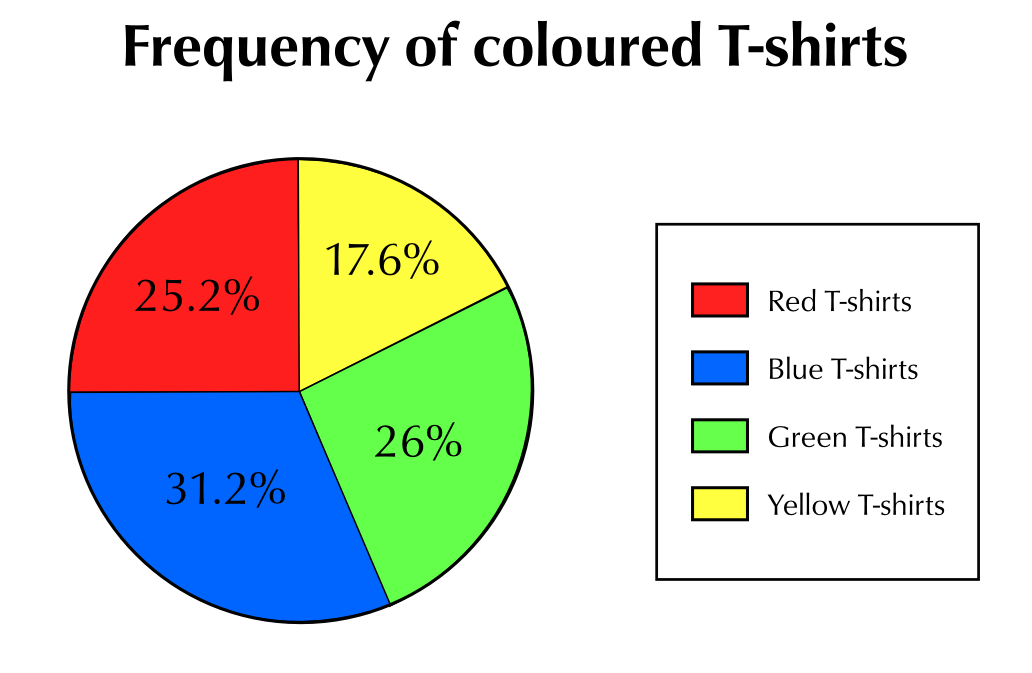The following list gives the weights of the learners in a class in kilograms.

$$\text{64}$$; $$\text{83}$$; $$\text{74}$$; $$\text{77}$$; $$\text{65}$$; $$\text{55}$$; $$\text{58}$$; $$\text{61}$$; $$\text{63}$$; $$\text{98}$$; $$\text{97}$$; $$\text{53}$$; $$\text{54}$$; $$\text{102}$$; $$\text{78}$$; $$\text{82}$$; $$\text{86}$$; $$\text{95}$$; $$\text{67}$$; $$\text{72}$$

Draw a frequency table to order the data, grouping it into $$\text{10}$$ $$\text{kg}$$ intervals.

 Interval (in kg) Frequency $$\text{50}$$ - $$\text{59}$$ $$\text{4}$$ $$\text{60}$$ - $$\text{69}$$ $$\text{5}$$ $$\text{70}$$ - $$\text{79}$$ $$\text{4}$$ $$\text{80}$$ - $$\text{89}$$ $$\text{3}$$ $$\text{90}$$ - $$\text{99}$$ $$\text{3}$$ $$\text{100}$$ - $$\text{109}$$ $$\text{1}$$

Use the frequency table to draw a histogram of the data.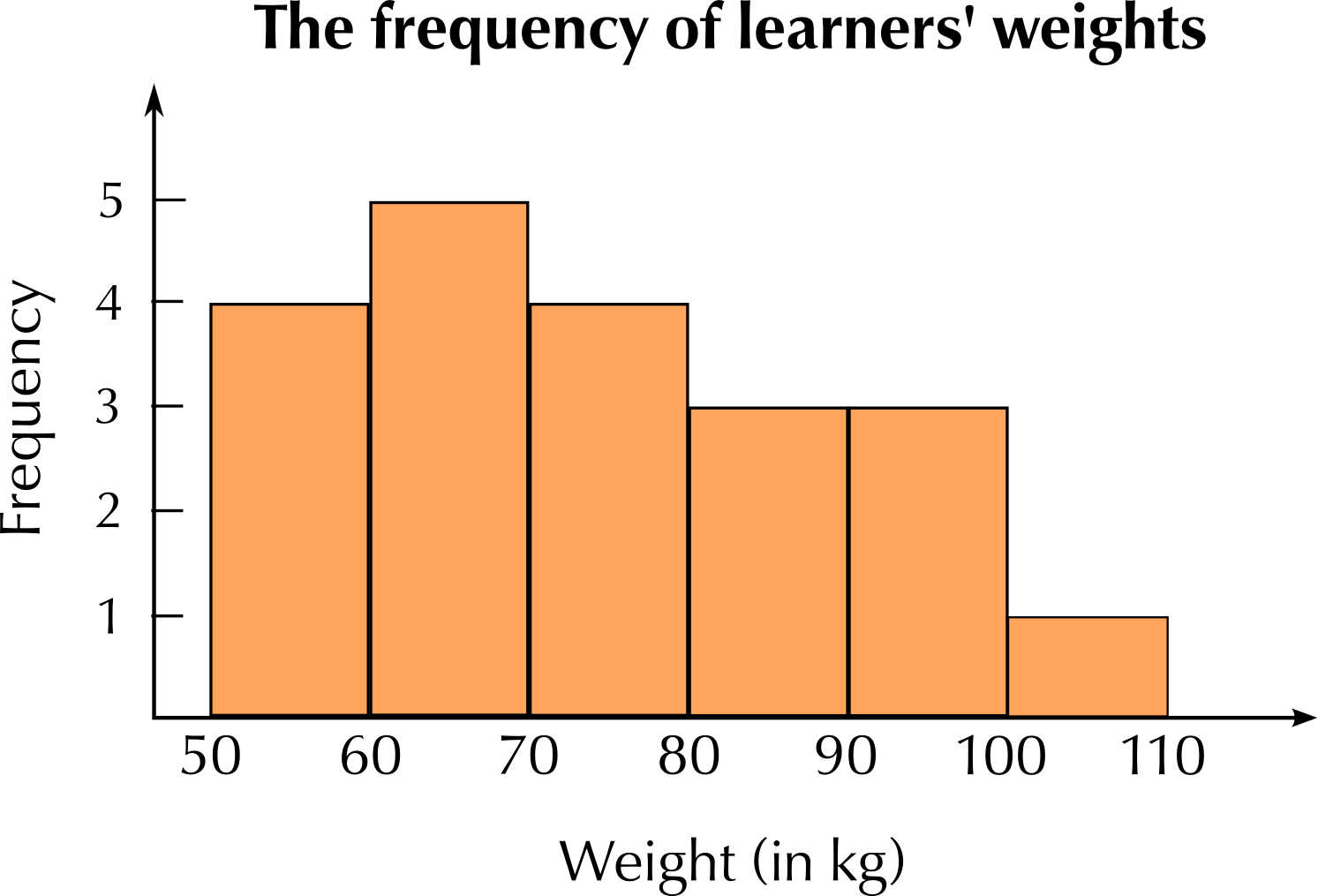The following table gives the maximum temperature (in °C) for each month in a year.

 Month Jan Feb Mar Apr May Jun Jul Aug Sep Oct Nov Dec Max Temp (°C) $$\text{27}$$ $$\text{28}$$ $$\text{25}$$ $$\text{21}$$ $$\text{20}$$ $$\text{17}$$ $$\text{18}$$ $$\text{19}$$ $$\text{20}$$ $$\text{24}$$ $$\text{25}$$ $$\text{27}$$

Draw a line graph of this data.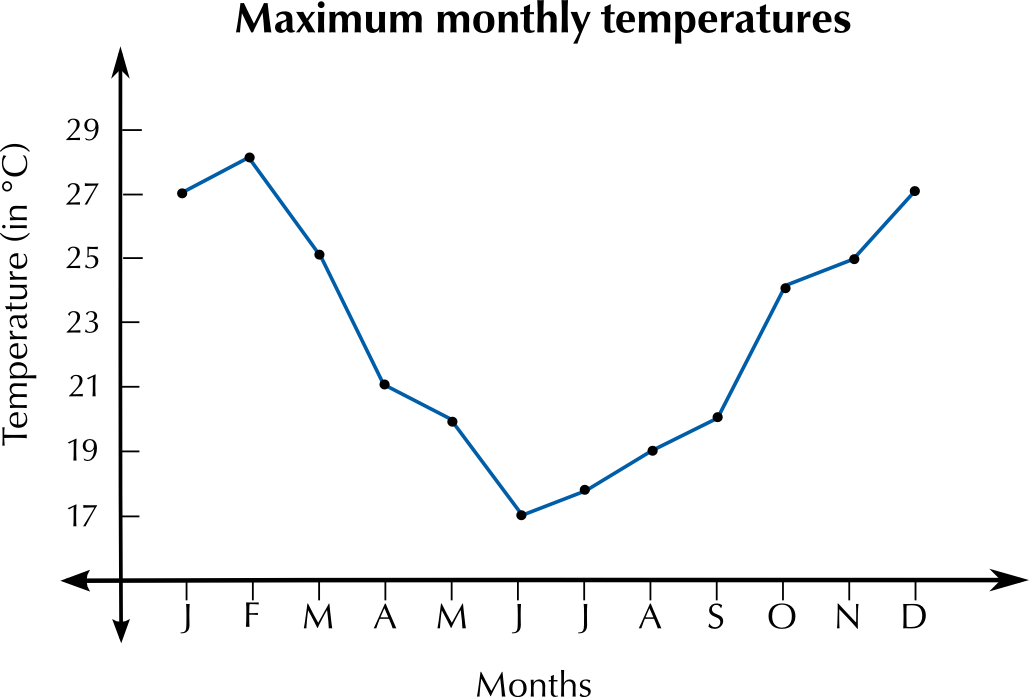Describe the trends you see in the data.

The maximum temperature drops towards the middle of the year, as we would expect during winter.

Answer the questions below about the following pie chart. The pie chart shows the favourite fruit juice flavours of a group of $$\text{120}$$ learners.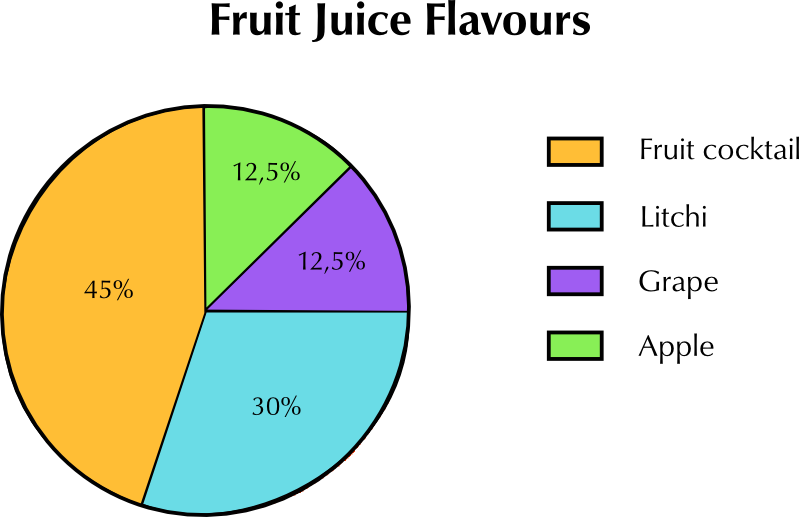Calculate how many learners chose each type of juice.

$$\text{45}\%$$ of $$\text{120}$$ learners = $$\text{54}$$ learners who chose fruit cocktail. $$\text{30}\%$$ of $$\text{120}$$ = $$\text{36}$$ learners who chose litchi. $$\text{12,5}\%$$ of $$\text{120}$$ learners = $$\text{15}$$ learners who chose grape. $$\text{12,5}\%$$ of $$\text{120}$$ = $$\text{15}$$ learners who chose apple juice.

In what way does the pie chart work better than a bar graph to represent this data?

The pie chart is a simple, visual representation that works well for representing percentages. A pie chart allows us to see at a glance the relative proportions of the learners who prefer each flavour.

What information would a bar graph give you that this pie chart does not?

The number of learners who prefer each flavour.

Look at the bar graph below and answer the questions that follow.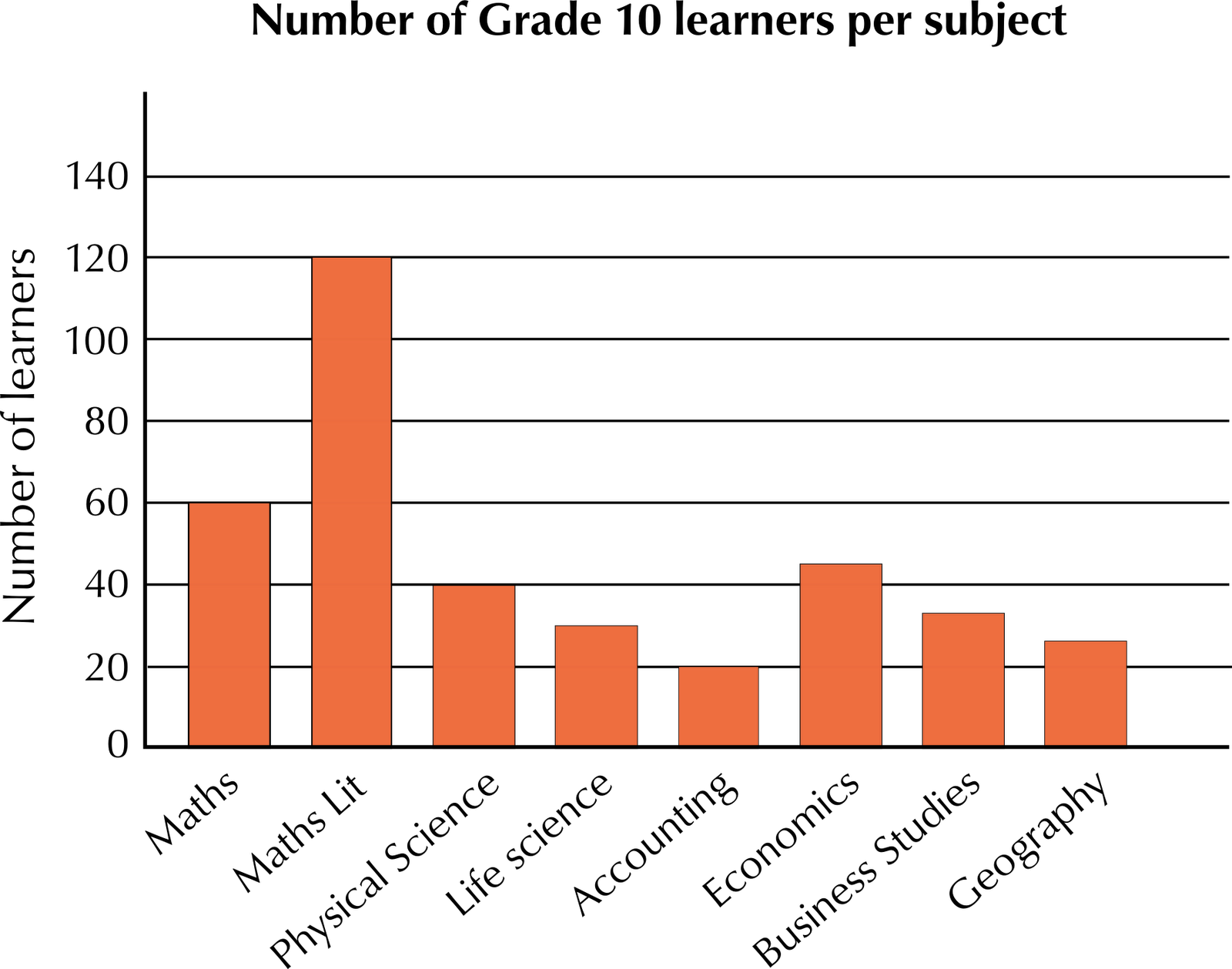Does this graph tell us how many Grade 10 learners there are in total?

No. It may look like there are $$\text{140}$$ learners in total but we have no way of knowing if that is correct or just an arbitrary number. Also, learners take more than one subject, so we can't use the numbers of learners per subject to determine how many learners there are altogether.

Can we assume that none of the learners who take Accounting take Geography?

No, we cannot assume this.

A pie graph of this data would not make sense. Explain why.

Learners do not only take one subject, therefore the data cannot be split into discrete percentages per subject and represented using a pie chart.